Push-pull stages are able to reduce harmonic distortions, specifically second order distortions, produced in the stage itself. The input stage has a single-ended configuration. A single-ended stage, operating in non-linear areas, produces distortions. If the distorted pre-amplified signal is given to a push-pull stage, distortions are amplified as well.

A technique to reduce distortions, across all amplifier stages, is the global negative feedback. Global negative feedback consists in using a negative feedback loop, which subtracts the output signal, appropriately attenuated, from the input signal.

The effect of the global negative feedback can be explained intuitively as follows. If the input signal and the output signal have identical shapes, the subtraction has the only effect of attenuating the input signal to be amplified and consequently the produced output signal. Similarly, when distortion signal is fed back, and subtracted from the input signal, distortion is also reduced. Suppose now we compare two output signals having the same level, one produced with global negative feedback and the other without. Note that distortion level depends on the output level, and does not depend on input level. This implies that, in both cases, the distortion internally introduced is the same. However, the outputs signal produced with negative feedback has less distortion, given that distortion itself has been attenuated through the feedback loop.

Figure 31 shows the basic schema of a global negative feedback loop. The feedback signal is produced by the voltage divider, composed of resistors R1 and R2, from the output signal. Resistor Ris generally called the feedback resistor. The feedback signal Vfb, provided by the voltage divider, is applied to the cathode of the input stage vacuum tube. When the output signal and the input signal have the same phase, this schema has the effect of subtracting the feedback signal Vfb from the input signal Vin. In fact, in this case, the feedback signal shifts the cathode voltage in the same direction of the input signal, reducing the grid to cathode voltage. In this way the signal at the grid of the input stage is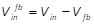. The feedback signal Vfb is obtained using the voltage divider equation:.

The factor βis generally referred as the feedback factor. The amount of feedback can be set by choosing β, by way of the voltage divider resistors.

Note that, if the phase of the output signal were inverted, with respect the input signal, the circuit would produce a positive feedback, which increases distortions and produces oscillations. When phase shifts are produced through the amplifier’s stages and affect the correct operation of the feedback loop, a capacitance Csn can be used, together with resistor R1, to form a step network, which has the purpose of maintaining a correct phase of the feedback signal at all relevant frequencies, guaranteeing stability of the amplifier. This is better discussed in Section 4.4.3.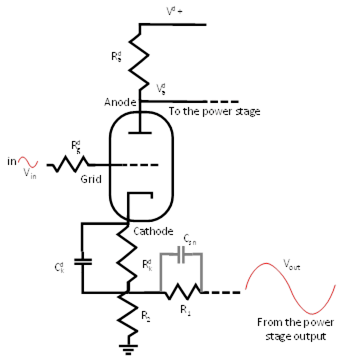Figure 31: Basic schema for global negative feedback.Global negative feedback is obtained by feeding the attenuated output signal back to the cathode of the input stage vacuum tube. If the output signal and the input signal have the same phase, the first is subtracted from the second, reducing distortions in the amplified signal. The voltage divider composed of the resistors R1 and R2 has the effect of attenuating the output voltage used as feedback. The capacitor Csn forms, with resistor R1, a step network to adjust the feedback signal phase to guarantee stability.

### 4.4.1    Gain with negative feedback

The gain of the amplifier without negative feedback is referred as the open loop gain of the amplifier. The open loop gain A is the ratio between the output and the input signals:.

We said that, when there is global negative feedback, the signal seen by the grid is. Therefore, the output signal is.

Simplifying we obtain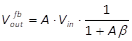.

The closed loop gain Afb of the amplifier, which is the gain of the amplifier when global negative feedback is used, can be obtained as the ratio between the output signal, with negative feedback loop, and the input signal:.

The quantity Aβ is generally referred as the loop gain, which is the gain seen in the feedback loop.

It is generally useful expressing the amount of negative feedback applied as the reduction of the gain in the amplifier. This can be easily obtained by expressing the gain in dB and computing the amount of feedback fbdB as: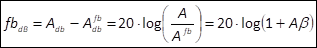.

For instance, if the gain of the amplifier without negative feedback is 20dB and the gain with global negative feedback is 14dB, we say that we apply fbdB=6dB of feedback.

### 4.4.2    Benefits of negative feedback

The use of negative feedbacks has several advantages. It stabilizes the gain of the amplifier, decreases output impedance, increases input impedance, increases bandwidth, and reduces distortions.

In the following, as an example, we discuss how negative feedback reduces harmonic distortions by a factor, at the same output level of the amplifier without negative feedback.

Suppose there is no negative feedback loop. In correspondence of the input signal Vin, the amplifier produces the output signal Vout + Vd, where Vd is the harmonic distortion introduced by the amplifier itself. The harmonic distortion percentage HD is measured as the ratio between the harmonic distortion signal and the output signal: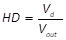Suppose now negative feedback loop is used and the input signal is increased from Vin to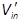, to compensate the gain loss and obtain an output signalequal to the output signal obtained without negative feedback. In other words, the increased input signalis such that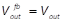. Now, the output of the amplifier, considering also distortions, is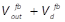. We can treat separately these two components using the equations discussed before: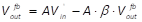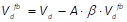.

The harmonic distortion Vd depends only on the output signal Vout (without negative feedback) or(with negative feedback). Given that we setequal to the output signal without feedback Vout, the harmonic distortion Vd, internally introduced by the amplifier, is the same in both cases. However, the second of the above equations says that when using negative feedback, Vd is also fed back and attenuated to. Simplifying, as before, we obtain that.

With negative feedback, the produced harmonic distortionis attenuated, with respect to the harmonic distortion Vd generated without negative feedback, at the same output level. For instance, a negative feedback fdB of 20 dB implies a closed loop gain 10 times lower than the open loop gain. However, harmonic distortion, at the same output level, will be ten times lower as well.

### 4.4.3    Stability of negative feedback

The schema for negative feedback, given in Figure 31, requires that the input signal and the negative feedback signal, applied to the cathode, have the same phase. However, when the input signal goes through the amplifier stages, its phase might shift significantly, reaching in some cases a 180° phase-shift. With a 180° phase-shift, the feedback signal has an opposite phase with respect to the input signal and the feedback circuit becomes a positive feedback circuit. Positive feedback is dangerous because it might introduce instability and oscillations.

Consider that both low-pass and high-pass filters produce a 45° phase-shift at their cut-off frequency and that phase-shifts are accumulated in a sequence of filters. Therefore, a 180° phase-shift becomes probable at frequencies near the borders of the bandwidth of the amplifier.

Suppose f180 is the frequency where the 180° phase-shift occurs, and A180 is the amplifier gain at this frequency. If the loop gain A180β is smaller than 1 (that is), positive feedback introduces just a gain peak at f180. However, if A180β is greater or equal to 1 (that is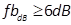), the amplifier will oscillate. In order to avoid this, the amplifier has to be designed so that the frequency, where the 180° phase-shifts occurs, is where A180β becomes smaller than 1. Low pass filters, obtained using grid stopper resistors, and high pass filters, produced by inter-stage coupling capacitors, can accomplish to this task.

However, not always low-pass and high-pass filters are able to effectively eliminate the conditions for oscillation and instability. In these cases, a step-network can be used to move the 180° phase-shift where A180β is smaller than 1. A step network is obtained by using a capacitor in parallel with the feedback resistor, as shown in Figure 31, with capacitor Csn and resistor R1.

 Example 15: Step network for negative feedback stability Consider, for instance, an amplifier with an open loop gain A=500 (54dB). Suppose that the solid and dashed red plot in Figure 32 respectively give the gain and phase-shift of the amplifier, with respect to the frequency. We can see that the amplifier introduces a phase-shift of -180°, around 240K Hz, where the gain A240KHz is still 25 (28dB). Suppose we apply a feedback factor β of 0.1, corresponding to a loop gain β∙A=0.1∙355=35.5 (fbdB=31.2dB), by setting R1 to 10K Ohm and R2 to 1K Ohm. At 240K Hz we have that the loop gain is β∙A240KHz=0.1∙25=2.5 (fbdB=10.9dB). Since the loop gain at 240K Hz is greater than 1 (fbdB>6dB) and the phase shift is -180°, the amplifier will oscillate. To avoid oscillation, we have to move the -180° phase-shift at a frequency f where the loop gain βAf is smaller than 1 (fbdB<6dB). To have βAf<1, with β=0.1, we need f such that the amplifier gain Afat frequency f is Af < 1/β=10 (20dB). The frequency where we have a 20dB gain is f=400K Hz. At this frequency, the phase-shift is -220°. To go below -180° we have to compensate at least 40°. Using a capacitor Csn of 80 pF, in parallel with R1, we obtain the frequency compensation shown by the red dashed line in Figure 33. We can see that the phase compensation at 240K Hz is 45° and at 400 Hz is 54°. The solid and dashed blue line in Figure 32 represents the loop gain and its phase-shift, with this compensation. At 240K Hz the phase-shift is now -135°, at 400K Hz it is -166°. The -180° phase-shift now occurs at 500K Hz, where the amplifier gain A500KHz is 5 (14 dB), thus below the threshold 10, which we identified before. The amplifier now is stable, in fact, βA500=0.1∙5=0.5, and fbdB=3.5dB.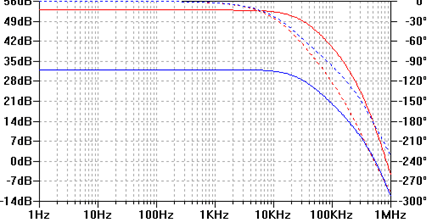Figure 32: Phase compensation for stable negative feedback.The gain and the phase-shift of the amplifier, with respect to the frequency, represented respectively by the red solid and dashed lines, show that we have a -180° phase-shift at 240K Hz, where the gain is still 28dB. Compensation, applied to the feedback loop, produces the loop gain and a phase-shift depicted by the blue solid and dashed lines. At 240K Hz, the phase-shift is 135°. 180° phase-shift is at 500K Hz, where amplifier gain is just 14dB.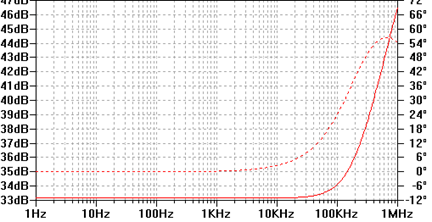Figure 33: Phase compensation with a step network.The step network, introduced in the feedback loop, produces the phase compensation depicted by the dashed line. We have a 45° compensation at 240K Hz and 54° at 400K Hz.